# KSEEB Solutions for Class 10 Maths Chapter 2 Triangles Ex 2.6

Students can Download Class 10 Maths Chapter 2 Triangles Ex 2.6 Questions and Answers, Notes Pdf, KSEEB Solutions for Class 10 Maths helps you to revise the complete Karnataka State Board Syllabus and score more marks in your in examinations

## Karnataka State Syllabus Class 10 Maths Chapter 2 Triangles Ex 2.6

Question 1.
In Fig. 2.56, PS is the bisector of ∠QPR of ∆ PQR, Prove that $$\frac{\mathbf{Q S}}{\mathbf{S R}}=\frac{\mathbf{P Q}}{\mathbf{P R}}$$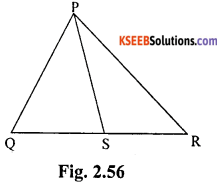Given: In the figure, PS is the bisector of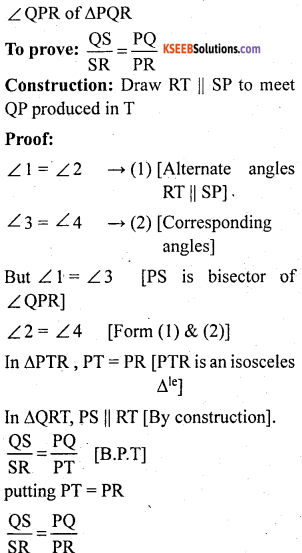Question 2.
In Fig. 2.57, D is a point on hypotenuse AD of ∆ ABC, such that BD ⊥ AC, DM ⊥ BC and DN ⊥ AB. Prove that:
i) DM2 = DN.MC ii) DN2 = DM.AN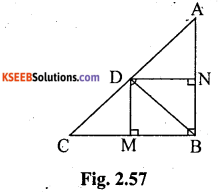Given: D is a point on hypotenuse AC of ∆ ABC DM ⊥ BC, DN ⊥AB and BD ⊥ ACii) Consider ∆le ADN And DBNQuestion 3.
In Fig. 2.58, ABC is a triangle in which ∠ABC > 90° and AD ⊥ CB produced. Prove that AC2 = AB2 + BC2 + 2BC. BD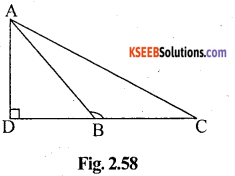Given: In figure, ABC is a triangle in which ∠ ABC > 90° and AD ⊥ BC.
To prove: AC2 = AB2 + BC2 + 2BC. BD.
Proof: In right triangle ABC
∠D = 90°
AC2 = AD2 + CD2 [Pythagoras theorem]
AC2 = AD2 + (BD + CB)2 [DC = BD + BC]
AC2 = AD2 + BD2+ BC2 +2.BD. BC
AC2 = AB2 + BC2 + 2BD.BC [In right angle ∆le ADB AB2 = AD2 + BD2]

Question 4.
In Fig. 2.59, ABC is a triangle in which ∠ ABC < 90° and AD ⊥ BC. Prove that AC2 = AB2 + BC2 – 2BC. BD.Given: In figure, ABC is a triangle in which ∠ABC < 90° and AD ⊥ BC
To prove: AC2 = AB2 + BC2 – 2BC.BD
Proof: In right triangle ABC ∠ D = 90°
AC2 = AD2 + DC2 [By pythagoras theorem]
= AD2 + (BC – BD)2 [CD = BC – BD]
= AD2 ± BC2 + BD2 – 2BC. BD
AC2 = AB2 + BC2 – 2BC.BD
∠D = 90° AB2 = AD2 + BD2 By Pythagoras theorem]Question 5.
In Fig. 2.60,AD is a median of a triangle ABC and AM I BC. Prove that: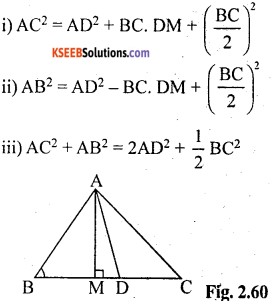Given: In figure, AD is a median of a triangle ABC and AM ⊥ BC.
∴ D is mid-point of BC (∵ AD is median)Proof:
i) In right triangle AMC ∠M = 90°
∴ AC2 = AM2 + MC2 [By Pythagoras theorem]
= AM2 +(MD + DC)2
= AM2 + MD2 + DC2 + 2MD. DC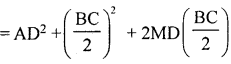[In right angle ∆le AMD with ∠M = 90°
AM2 + MD2 = AD2 (By pythagoras theorem)]ii) In right triangle AMB ∠M = 90°
AB2 = AM2 + MB2 [Pythagoras
theorem]
= AM2 + (BD – MD)2
= AM2 + BD2 + MD2 – 2BD . MD
= AM2 + MD2 + BD2 – (2 BD)MD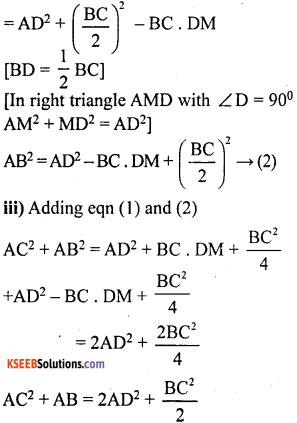Question 6.
Prove that the sum of the squares of the diagonals of parallelogram is equal to the sum of the squares of its sides.Given: In parallelogram ABCD
AB = CD & AD = BC
Construction: convert parallelogram into a rectangle and Draw AG ⊥ CD
To prove:
AC2 + BD2 = AB2 + BC22 + CD2 – AD2
Proof: consider ∆le BDF ∠D = 90°
BD2 = BF2 + FD2 = h2 + (x+d)2 — (1)
Consider ∆le AGC ∠G = 90°
AC2 = AG22 + GC2 = h2 + (x – d)2 — (2)
AC2+ BD2 = h2 + (x + d)2 + h2 + (x – d)2
2h2 + x2 + 2xd + d2 + x2 – 2xd + d2
2h2 + 2x2 + 2d2
= 2x2 + 2(h2 + d2)
= 2x2 + 2y2
= x2 + y2 + x2 + AC2 + BD2 =AB2 +BC2 i-CD2 + AD2.

Question 7.
In Fig. 2.61, two chords AB and CD intersect each other at the point P.
Prove that:
i) ∆ APC ~ ∆ DPB
ii) AP. PB = CP.DP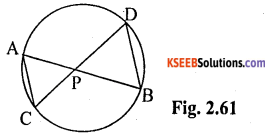Given: In figure, two chords AB and CD intersect each other at the point P.
To prove: i) ∆ APC ~ ∆ DPB
ii) AP. PB = CP . DP
Proof: i) In ∆ APC and ∆ DPB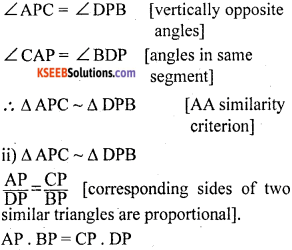Question 8.
In Fig. 2.62, two chords AB and CD of a circle intersect each other at the point P (when produced) outside the circle. Prove thatGiven: In figure, two chords AB and CD of a circle intersect each other at the point P. (when produced) outside the circle.
To prove: i) ∆ PAC ~ ∆ PDB
ii )PA. PB = PC . PD
Proof: i) we know that in a cyclic quadrilateral, the exterior angle is equal to the interior opposite angle.
consider ABCD ∆ PAC and ∆ PBD.
∠PAC = ∠PDB → (i)
∠PCA = ∠PBD → (2)
∆ PAC ~ ∆ PDB [A A similarity criterion]

ii) A PAC ~ ∆ PDB (Proved)
$$\frac{P A}{P D}=\frac{P C}{P B}$$ [Corresponding sides of the similar ∆le are proportional]
PA . PB = PC . PDQuestion 9.
In Fig. 2.63, D is a point on side BC of ∆ ABC such that $$\frac{B D}{C D}=\frac{A B}{A C}$$ Prove that AD is the bisector of ∠BAC.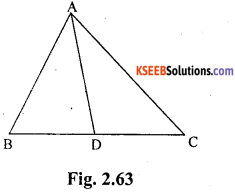Given: In figure, D is a point on side BC. of ∆ ABC such that $$\frac{B D}{C D}=\frac{A B}{A C}$$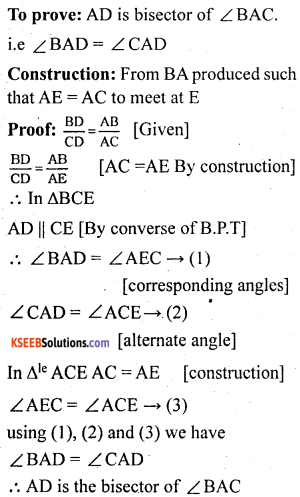Question 10.
Nazima is fly fishing in a stream. The tip of her fishing rod ¡s 1.8 m above the surface of the water and the fly at the end of the string rests on the water 3.6 m away nad 2.4 m from a point directly under the tip of the rod. Assuming that her string (from the tip of her rod to the fly) is taut, how much string does she have out (see Fig. 2.64)?If she pulls in the string at the rate of 5 cm per second, what will be the horizontal distance of the fly from her after 12 seconds?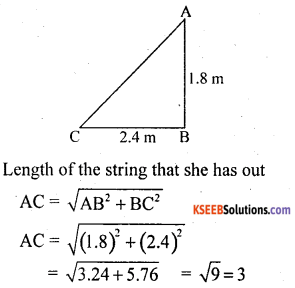AC = 3m
Hence, she has 3 m string out.
Length of the string pulled in 12 seconds
at the rate of5 cm/ sec 5 × 12cm = 60 cm = O.6 m.
∴ Length of remaining string left out
= AD = 3.0 – 0.6 = 2.4mIn right angled ∆ ABD ∠B = 90°